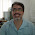## Wednesday, March 27, 2013

### Why large N is useful

In Hewson's book The Kondo problem to heavy fermions he devotes two whole chapters (7 and 8) to large N limits.

This is a powerful technique in quantum many-body theory and statistical physics. One expands the continuous symmetry of a specific model (e.g. from SU(2) to SU(N)) and then takes the limit of large N. If coupling constants are scaled appropriately the limit of infinite N can be solved analytically by a mean-field theory. One then considers corrections in powers of 1/N. This idea was first pursued for classical critical phenomena where the order parameter was an N-dimensional vector and the symmetry was O(N).

In the Kondo problem this is a particularly powerful and important technique for several reasons.

First, large N can be physically relevant. For example, cerium impurities have N=6 (N=14) when spin-orbit coupling is (not) taken into account.

Second, for any N there are exact results for thermodynamic properties from the Bethe ansatz solution. These provide something to benchmark approximate results from large N treatments.

Third, one can obtain results for dynamical and transport properties which cannot be calculated with the Bethe ansatz.

Fourth, because the analytics/mathematics is relatively simple in the slave boson and diagrammatic formulations one can gain some insight as to what is going on (perhaps).

Fifth, the large N limit and slave bosons can also be used to study lattice models such as Hubbard and Kondo lattice models.

Finally, it works!, giving results that are better both qualitatively and quantitatively than one might expect. For example, Bickers, Cox, and Wilkins used large N to give a comprehensive description of a whole range of experimental properties.

But, the slave boson mean-field theory is far from perfect, working best below the Kondo temperature. In particular, it produces an artifact: a finite temperature phase transition (Bose condensation) at a temperature comparable to the Kondo temperature.

#### 2 comments:

1.Hi Ross,

I would add on the "plus" side the fact that the large-N limit is often a cheap way to have access to non-perturbative physics, as is the case in the Kondo problem, which is quite hard otherwise.

Cheers,
Eduardo Miranda.

1.Hi Eduardo,

Thanks for the helpful comment. I agree. The "cheapness" of the large N limit is certainly one of its big selling points.

cheers
Ross